## How to Graph Single Variable Inequalities?

### Steps to graphing single–variable inequalities

• Inequality is comparable to equations and employs symbols such as “less than” ($$<$$) and “greater than” ($$>$$).
• To find the answer for inequalities, you must isolate the variable. (as in equations)
• To graph the inequality, figure out its value on a number line.
• For less than or greater than you need to draw an open circle on the variable’s value.
• If it also has an equal sign, you must then use a filled circle.
• Put a line to the right or to left for greater or less than.

To understand how to graph inequalities better, below are some examples

Example one:
Make a graph for $$x > 5$$.
As the variable is higher than $$5$$, you must find $$5$$ and draw an open circle above it. Afterward, draw a line to the right.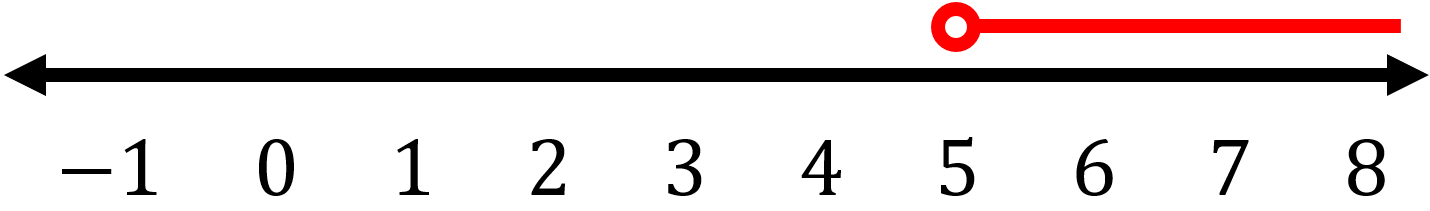Example two:
Make a graph for $$x \ < \ 4$$.
As the variable is lower than $$4$$, you must find $$4$$ and then draw an open circle above it. Afterward, draw a line to the left.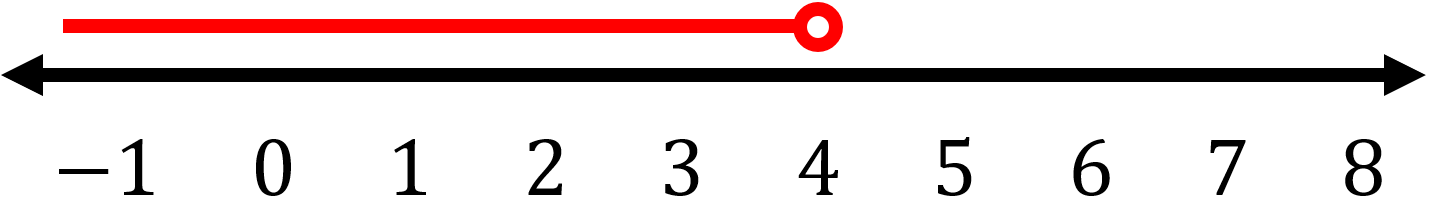### Exercises for Graphing Single Variable Inequalities

1) Make a graph for $$x \ > \ -2$$

2) Make a graph for $$x \ ≤ \ 1$$

3) Make a graph for $$x \ + \ 3 \ ≥ \ -2$$

4) Make a graph for $$x \ - \ 9 \ ≤ \ -10$$

5) Make a graph for $$x \ > \ -4$$

6) Make a graph for $$x \ < \ 5$$

7) Make a graph for $$x \ - \ 6 \ < \ -3$$

8) Make a graph for $$x \ - \ 7 \ < \ -5$$

9) Make a graph for $$x \ + \ 11 \ > \ 13$$

10) Make a graph for $$x \ - \ 6 \ ≤ \ -9$$

1) Make a graph for $$x \ > \ -2$$

Since the variable ($$x$$) is greater than $$3$$, first, we should find $$3$$, then draw an open circle above it and draw a line to the right.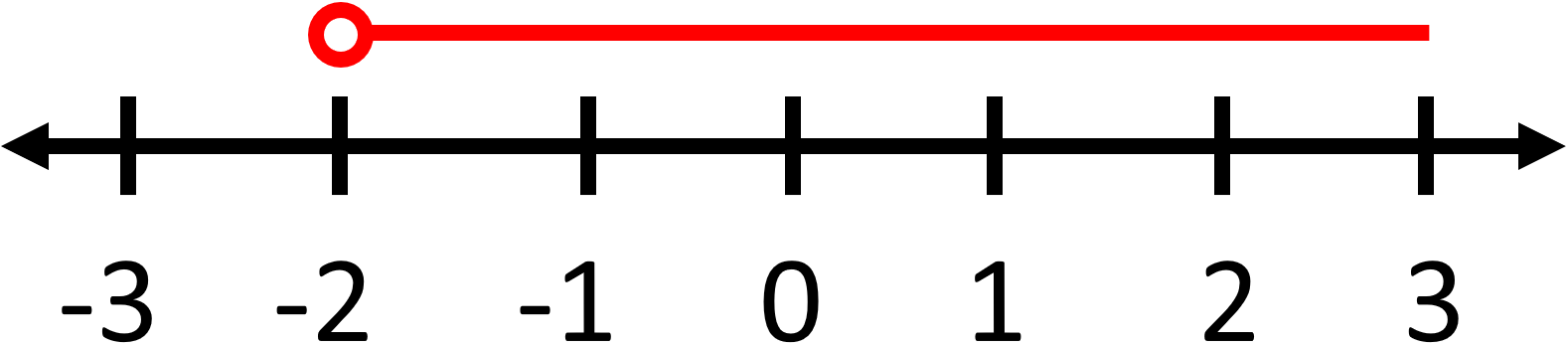2) Make a graph for $$x \ ≤ \ 1$$

As the variable is lower than or equal to $$1$$, you must find $$1$$ and then draw a close circle above it. Afterward, draw a line to the left.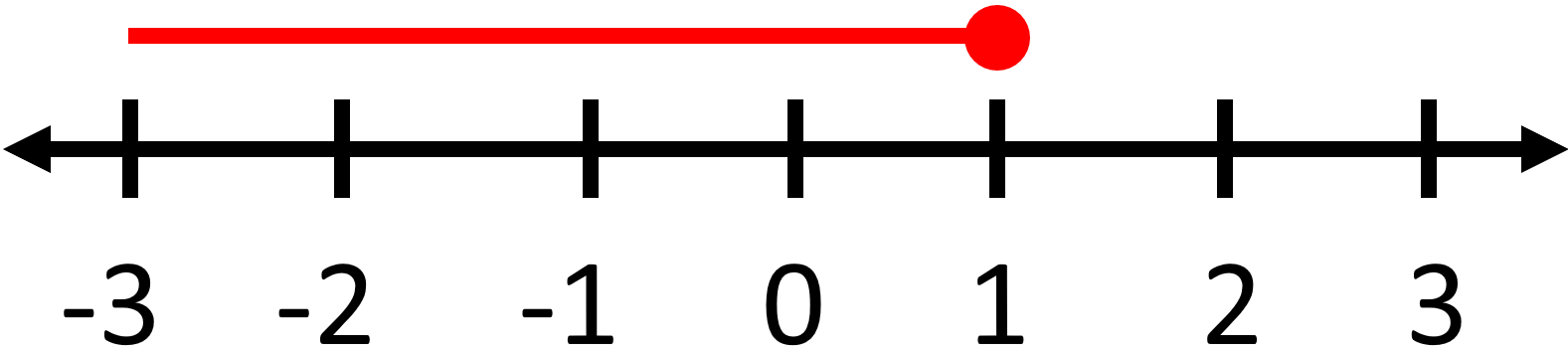3) Make a graph for $$x \ + \ 3 \ ≥ \ -2$$

In this example first, we must isolate the variable. To do this subtract $$3$$ from both sides:
$$x \ + \ 3 \ - \ 3 \ ≥ \ -2 \ - \ 3 \ ⇒ \ x \ ≥ \ -5$$
Now, Since the variable ($$x$$) is greater than or equal to $$-5$$, we must find $$-5$$ and then draw a close circle above it. Afterward, draw a line to the right.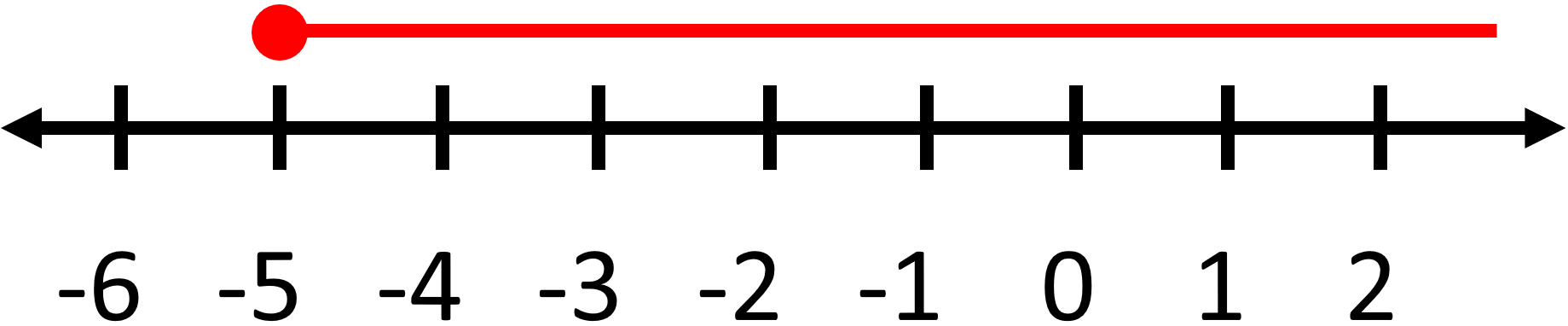4) Make a graph for $$x \ - \ 9 \ ≤ \ -10$$

In this example first, we must isolate the variable. To do this add $$9$$ to both sides:
$$x \ - \ 9 \ + \ 9 \ ≤ \ -10 \ + \ 9 \ ⇒ \ x \ ≤ \ -1$$
Now, Since the variable ($$x$$) is lower than or equal to $$-1$$, we must find $$-1$$ and then draw a close circle above it. Afterward, draw a line to the left.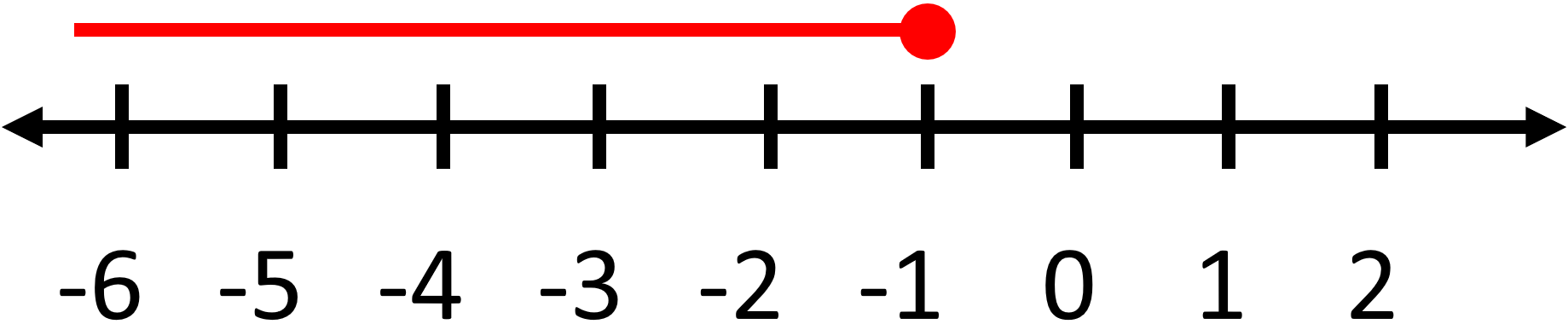5) Make a graph for $$x \ > \ -4$$

Since the variable ($$x$$) is greater than $$-4$$, first, we should find $$-4$$, then draw an open circle above it and draw a line to the right.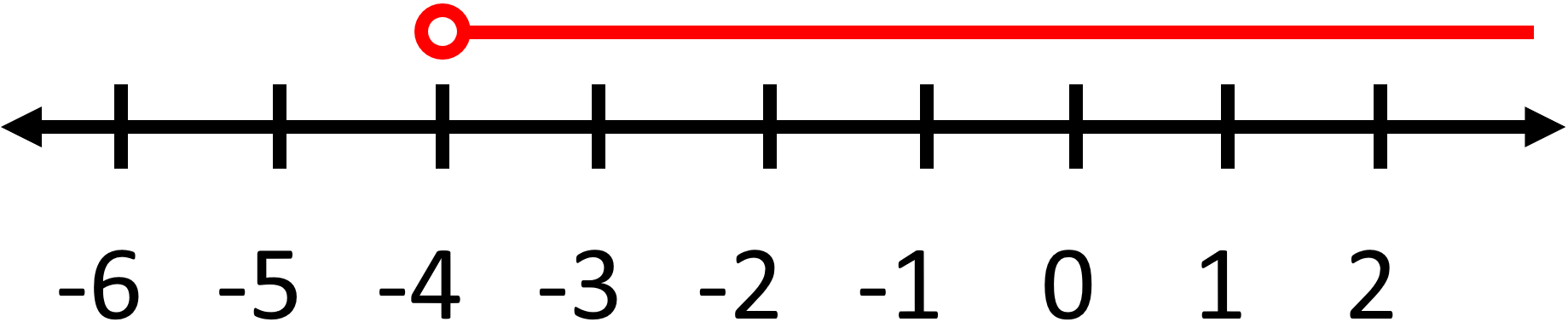6) Make a graph for $$x \ < \ 5$$

As the variable is lower than $$5$$, you must find $$5$$ and then draw an open circle above it. Afterward, draw a line to the left.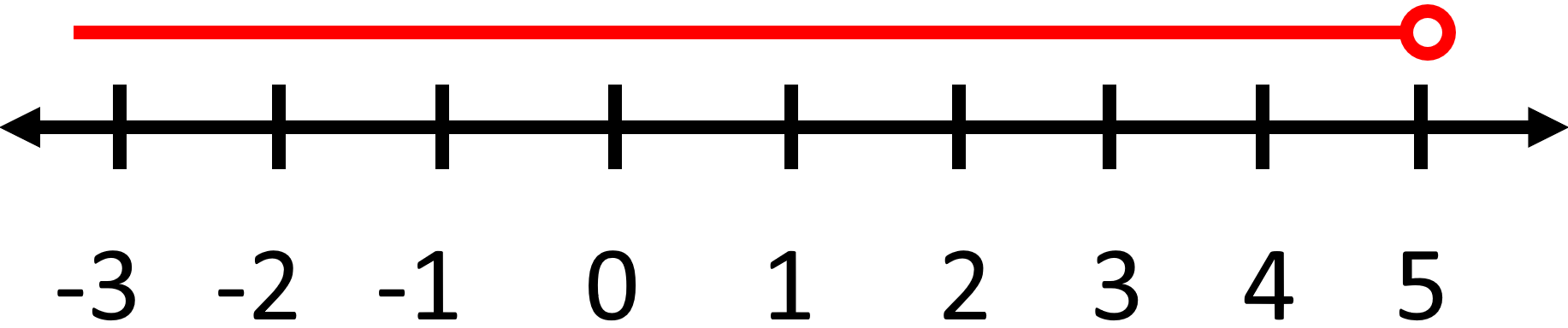7) Make a graph for $$x \ - \ 6 \ < \ -3$$
In this example first, we must isolate the variable. To do this add $$6$$ to both sides:
$$x \ - \ 6 \ + \ 6 \ < \ -3 \ + \ 6 \ ⇒ \ x \ < \ 3$$
Now, Since the variable ($$x$$) is lower than $$3$$, we must find $$3$$ and then draw an open circle above it. Afterward, draw a line to the left.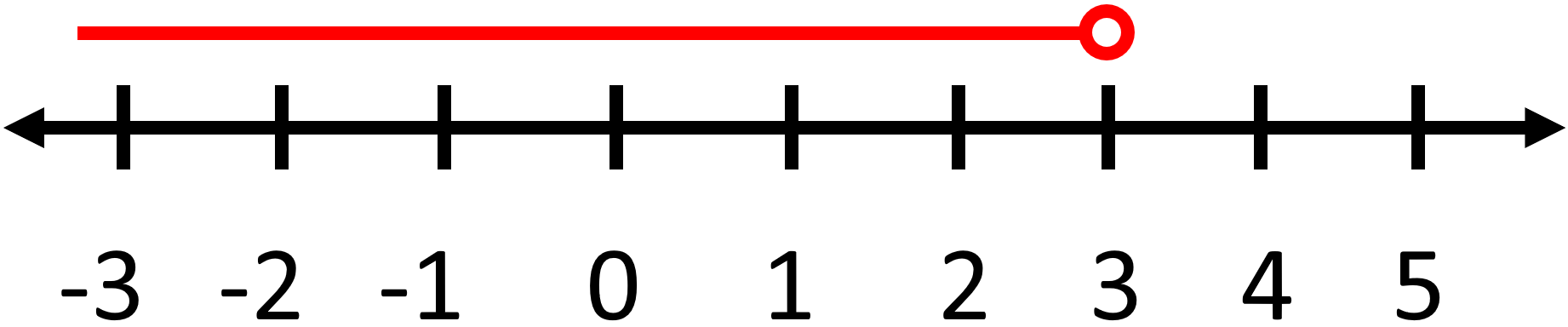8) Make a graph for $$x \ - \ 7 \ < \ -5$$
In this example first, we must isolate the variable. To do this add $$7$$ to both sides:
$$x \ - \ 7 \ + \ 7 \ < \ -5 \ + \ 7 \ ⇒ \ x \ < \ 2$$
Now, Since the variable ($$x$$) is lower than $$2$$, we must find $$2$$ and then draw an open circle above it. Afterward, draw a line to the left.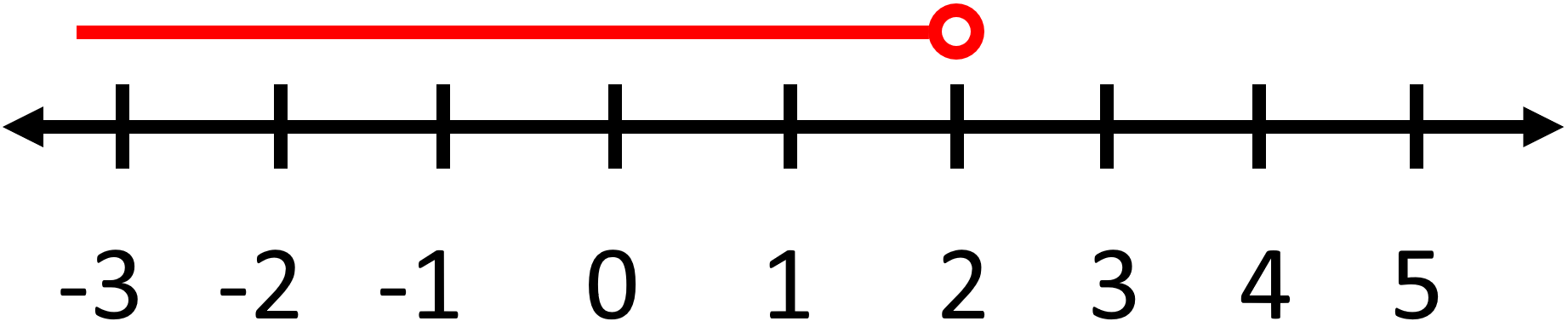9) Make a graph for $$x \ + \ 11 \ > \ 13$$
In this example first, we must isolate the variable. To do this subtract $$11$$ from both sides:
$$x \ + \ 11 \ - \ 11 \ > \ 13 \ - \ 11 \ ⇒ \ x \ > \ 2$$
Now, Since the variable ($$x$$) is greater than $$2$$, we must find $$2$$ and then draw an open circle above it. Afterward, draw a line to the right.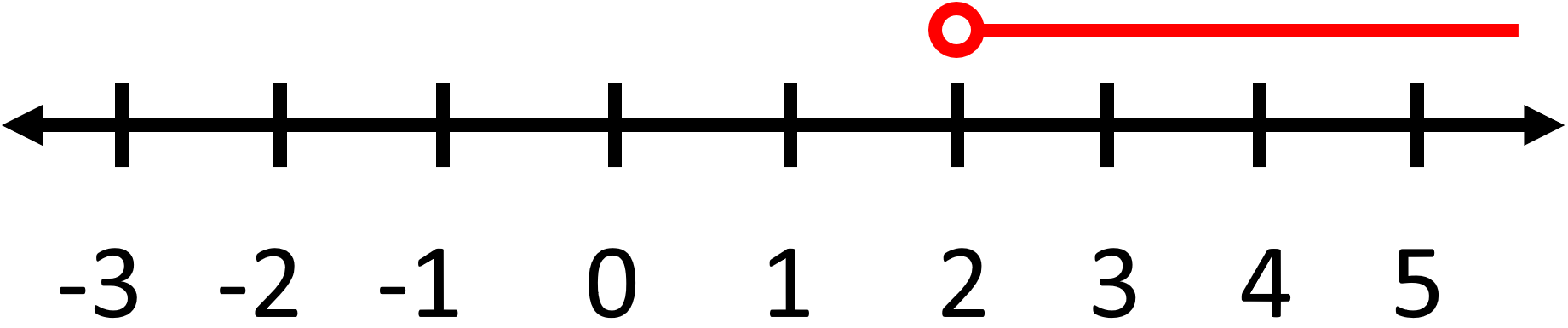10) Make a graph for $$x \ - \ 6 \ ≤ \ -9$$
In this example first, we must isolate the variable. To do this add $$6$$ to both sides:
$$x \ - \ 6 \ + \ 6 \ ≤ \ -9 \ + \ 6 \ ⇒ \ x \ ≤ \ -3$$
Now, Since the variable ($$x$$) is lower than or equal to $$-3$$, we must find $$-3$$ and then draw a close circle above it. Afterward, draw a line to the right.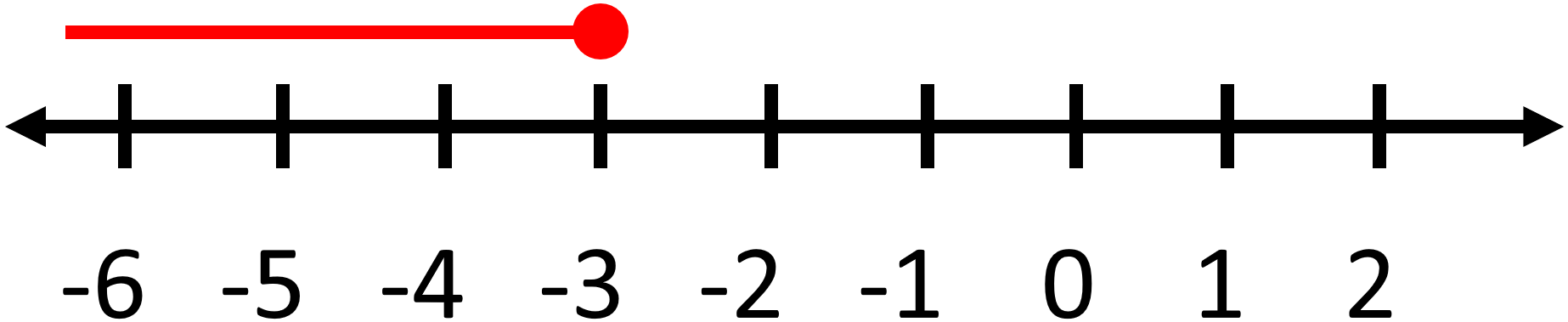## Graphing Single Variable Inequalities Quiz

### GED Mathematical Reasoning Formulas

$6.99$5.99

### TASC Math for Beginners

$24.99$14.99

### CLEP College Mathematics Practice Workbook

$25.99$14.99

### TABE 11 & 12 Math Study Guide

$20.99$15.99